# Worksheet Chapter 15 (2008) - Worksheet Chapter 15 Define...

• Notes
• 8
• 100% (2) 2 out of 2 people found this document helpful

This preview shows page 1 - 3 out of 8 pages.

The preview shows page 1 - 3 out of 8 pages.
WorksheetChapter 15Define the following:A.chemical equilibrium-B.law of mass action-C.Le Chatelier's principle-D.heterogeneous equilibriaSolve the following:1.What is a heterogeneous catalyst? ___________________________2.What is the equilibrium expression for the following reaction:2A+5B___________________3.What is the Kpof the above reaction if the PA= 35 torrs, PB= 10torrs, and PC= 0.56 torrs?3C______________4.What is the equilibrium expressions for Kc and Kp for the followingreaction?SnO2(s)+2CO(g)Sn(s)+2CO2(g)_____________________________, ___________________________5.What is the equilibrium constant for the above equation if theequilibrium concentrations are:[CO] = 3.4 M, [CO2] = 2.1 x 10-1_______________6.What is the direction of the equilibrium of Number 5? _________7.A mixture of 1.374 g of H2, and 70.31 g of Br2is heated in aM
##### We have textbook solutions for you!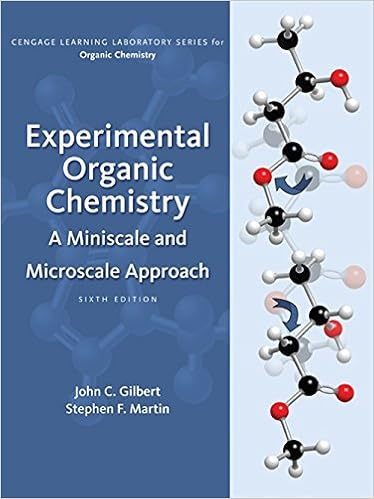The document you are viewing contains questions related to this textbook.
Chapter 18 / Exercise 10
Experimental Organic Chemistry: A Miniscale & Microscale Approach
Gilbert/MartinExpert Verified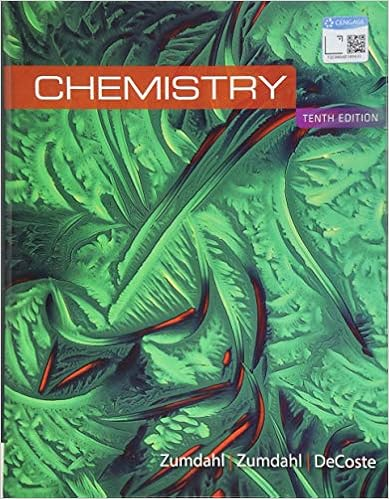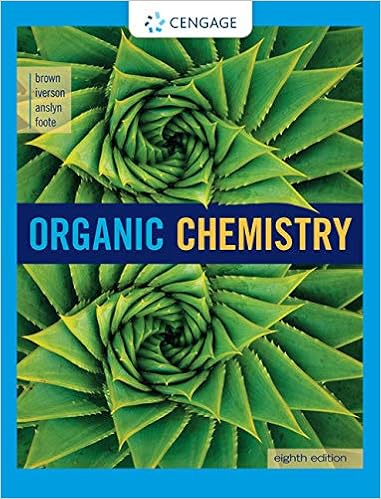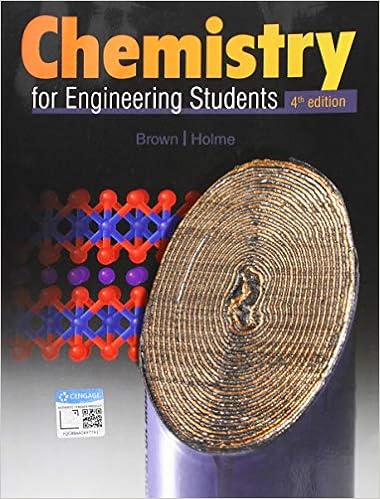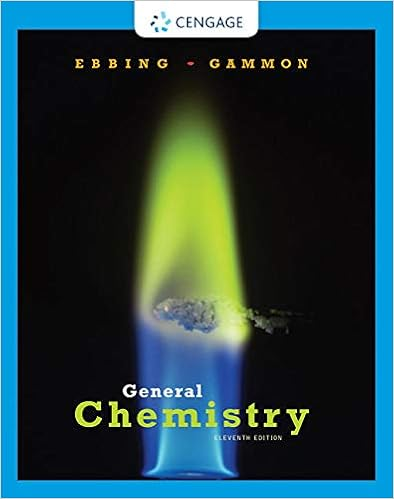Browse all Textbook Solutions
2.00 L vessel at 700 K.These substances react as follows:H2(g)+Br2(g)2HBr(g)At equilibrium the vessel is found to contain 0.566 g of H2.(a)Calculate the equilibrium concentrations of H2, Br2, and HBr.(b)Calculate Kc.__________8.For problem 7, what is the Kcfor the reverse reaction?_______________9.At 373 K, Kp = 0.416 for the equilibrium2NOBr(g)

Course Hero member to access this document

Course Hero member to access this document

End of preview. Want to read all 8 pages?

Course Hero member to access this document

Term
Spring
Professor
Deeter,G
Tags
##### We have textbook solutions for you!
The document you are viewing contains questions related to this textbook.The document you are viewing contains questions related to this textbook.
Chapter 18 / Exercise 10
Experimental Organic Chemistry: A Miniscale & Microscale Approach
Gilbert/MartinExpert Verified
•••